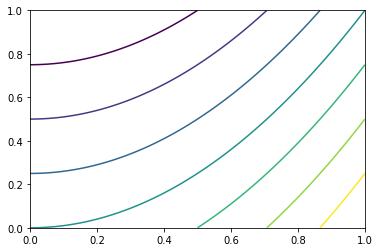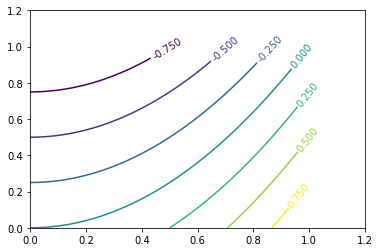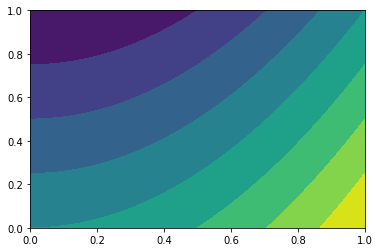# Contour plots

Contour plots allow us to project a third dimension of data down onto an X, Y axis, be it a functional relationship between two dimensions of data or some decision threshold of a given model.

But before we can start plotting this third dimension of data, we have to figure out how to calculate it.

## Meshgrid

Say we’ve got two simple vectors, x and y.

%pylab inline

x = np.linspace(0, 10, 5)
y = np.linspace(0, 1, 4)
Populating the interactive namespace from numpy and matplotlib


x has length 5 and y has length 4

print(x)
print(x.shape)
print(y)
print(y.shape)
[  0.    2.5   5.    7.5  10. ]
(5,)
[ 0.          0.33333333  0.66666667  1.        ]
(4,)


We want to calculate a value for a third vector z ** at every point these two meet**, therefore, we need to express z with dimensions (len(x), len(y)).

This is where meshgrid() comes in.

xx, yy = np.meshgrid(x, y)

It produces a matrix xx where our original vector x is broadcast as each row, with the same number of rows as y has length.

print(xx)
print(xx.shape)
[[  0.    2.5   5.    7.5  10. ]
[  0.    2.5   5.    7.5  10. ]
[  0.    2.5   5.    7.5  10. ]
[  0.    2.5   5.    7.5  10. ]]
(4, 5)


Similarly, yy has each column as y vectors, with as many columns as there are x values.

print(yy)
print(yy.shape)
[[ 0.          0.          0.          0.          0.        ]
[ 0.33333333  0.33333333  0.33333333  0.33333333  0.33333333]
[ 0.66666667  0.66666667  0.66666667  0.66666667  0.66666667]
[ 1.          1.          1.          1.          1.        ]]
(4, 5)


Now because we have two matricies of the same dimensions, we can do element-wise calculations and preserve our dimensions.

z = xx + yy
z.shape
(4, 5)

z = xx ** yy
z.shape
(4, 5)

z = xx > yy
z.shape
(4, 5)


## Contour

Now that we know how to get clean values for Z, generating a contour plot is a simple call to plt.contour()

X = np.linspace(0, 1, 1000)
Y = np.linspace(0, 1, 1000)

xx, yy = np.meshgrid(X, Y)

Z = xx ** 2 - yy 

Ez pz

plt.contour(X, Y, Z)
<matplotlib.contour.QuadContourSet at 0x1f8bf254eb8>### Labelling Lines

Labelling the contours is also pretty simple. You may have noticed that the call to contour() returned a matplotlib.contour.QuadContourSet object. If we catch this, we can pass it as an argument to ax.clabel()

fig, ax = plt.subplots()
cs = ax.contour(X, Y, Z)
ax.clabel(cs)

# adjusting axis because my X, Y were
# pooly-chosen, lol
ax.set_xlim(0, 1.2)
ax.set_ylim(0, 1.2)
(0, 1.2)### Filled Contour

Filling in the contours is just as simple– merely tacking an f onto the function name!

plt.contourf(X, Y, Z)
<matplotlib.contour.QuadContourSet at 0x1f8bf5f36d8>